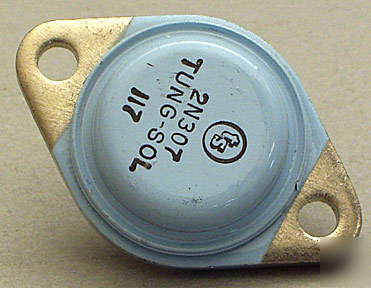Oak Bluffs, MA old classifieds archive and funnies   >   Industrial Machines   >   2N307 transistor germanium pnp power bjt tung-sol to-3

## 2N307 transistor germanium pnp power bjt tung-sol to-3

2N307 Transistor Germanium PNP Power BJT Tung-Sol TO-3
Bipolar germanium power PNP transistor, type 2N307. Made by Tung-Sol, in good condition.
Description = Ge PNP Power BJT
I(C) Abs.(A) Collector Current = 5.0
Absolute Max. Power Diss. (W) = 106
@V(CBO) (V) (Test Condition) = 25
@I(C) (A) (Test Condition) = 200m
@I(B) (A) (Test Condition) = 20m
h(FE) Min. Static Current Gain = 20
@I(C) (A) (Test Condition) = 200m
@V(CE) (V) (Test Condition) = 1.0
f(T) Min. (Hz) Transition Freq = 3.0k
@I(C) (A) (Test Condition) = 1.0
@V(CE) (V) (Test Condition) = 6.0